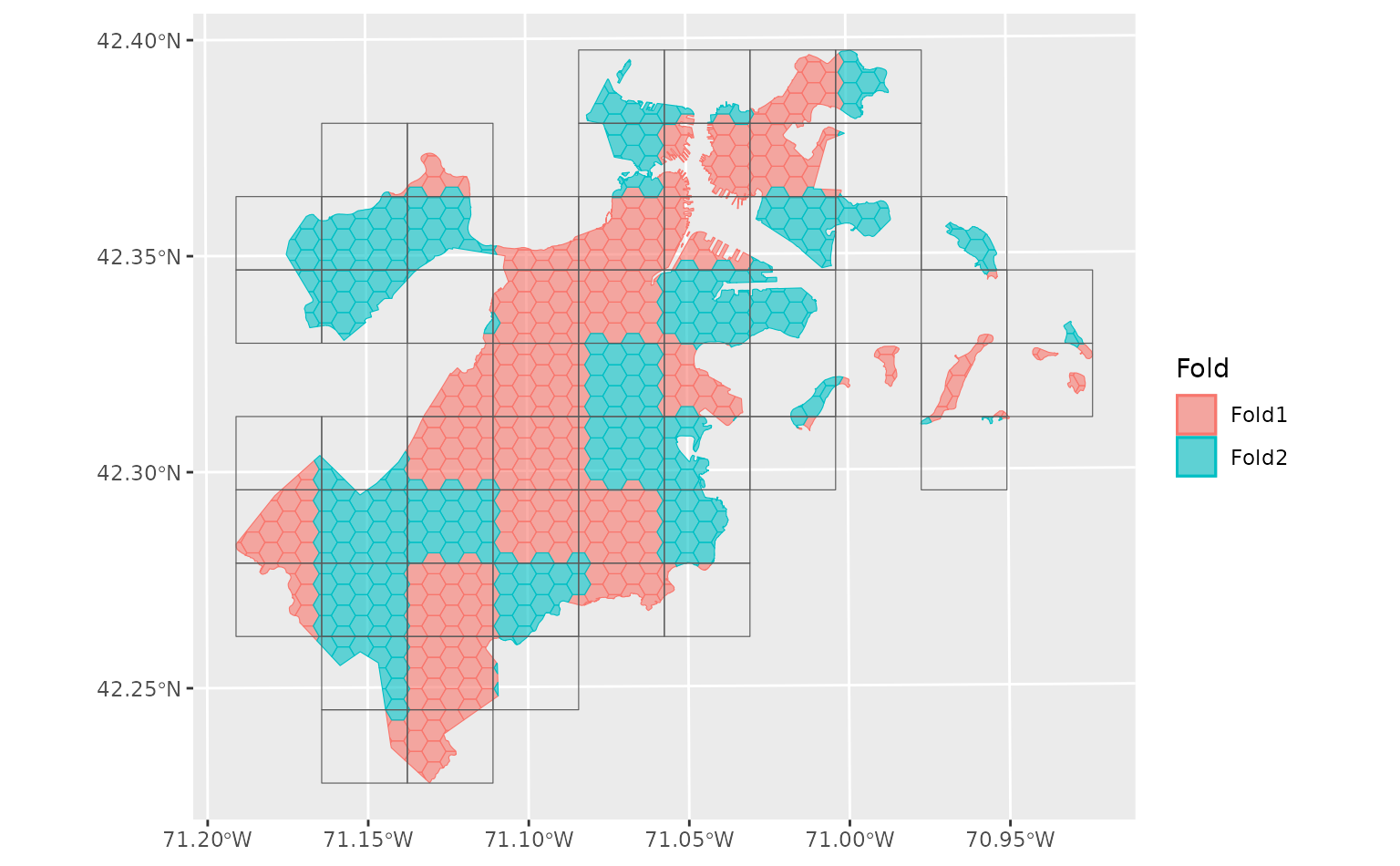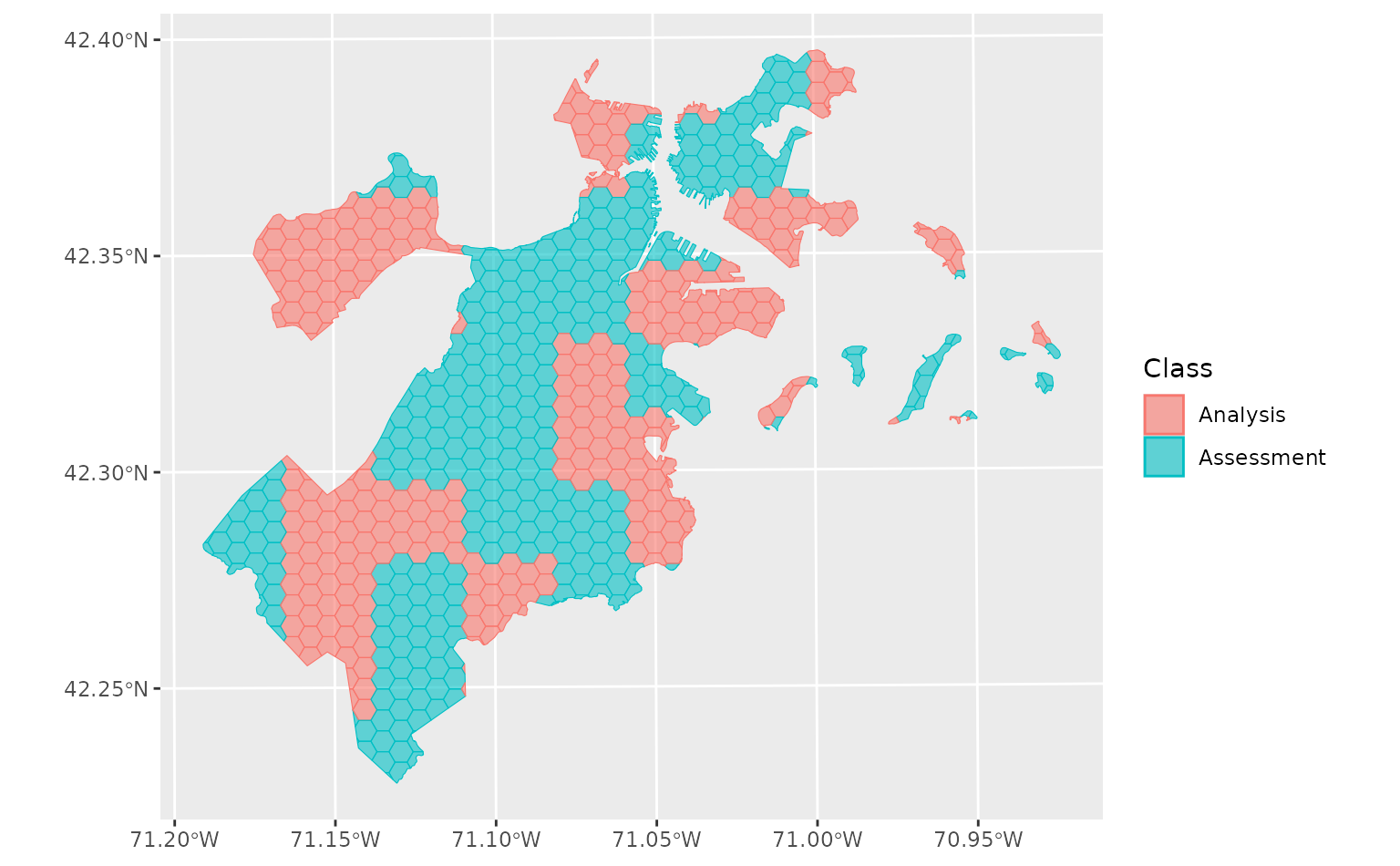This method provides a good visualization method for spatial resampling.

## Usage

# S3 method for spatial_rset
autoplot(object, ..., alpha = 0.6)

# S3 method for spatial_block_cv
autoplot(object, show_grid = TRUE, ..., alpha = 0.6)

## Arguments

object

A spatial_rset object or a spatial_rsplit object. Note that only resamples made from sf objects create spatial_rset and spatial_rsplit objects; this function will not work for resamples made with non-spatial tibbles or data.frames.

...

Options passed to ggplot2::geom_sf().

alpha

Opacity, passed to ggplot2::geom_sf(). Values of alpha range from 0 to 1, with lower values corresponding to more transparent colors.

show_grid

When plotting spatial_block_cv objects, should the grid itself be drawn on top of the data? Set to FALSE to remove the grid.

## Value

A ggplot object with each fold assigned a color, made using ggplot2::geom_sf().

The plot method for spatial_rset displays which fold each observation is assigned to. Note that if data is assigned to multiple folds (which is common if resamples were created with a non-zero radius) only the "last" fold for each observation will appear on the plot. Consider adding ggplot2::facet_wrap(~ fold) to visualize all members of each fold separately. Alternatively, consider plotting each split using the spatial_rsplit method (for example, via lapply(object$splits, autoplot)). ## Examples  boston_block <- spatial_block_cv(boston_canopy, v = 2) autoplot(boston_block)autoplot(boston_block$splits[])